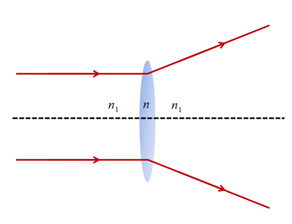# Ray Optics MCQs for NEET

NEET  Physics is the scoring paper in the medical entrance examination. Here, you will discover the NEET Physics MCQ Questions for all Concepts as per the latest syllabus. Practice more on a regular basis with these NEET Physics objective questions on air pollution and improve your subject knowledge & problem-solving skills along with time management. NEET Physics Ray Optics Multiple Choice Questions make you feel confident in answering the question in the exam & increases your scores to high.

## MCQ on Ray Optics

1. What is the distance between two convex lenses LA and LB with focal lengths FA and FB?
(a) FA+FB
(b) FA-FB
(c) FA
(d) FB

2. If a medium has a critical angle for total internal reflection from the medium to vacuum as 300, what is the velocity of light in the medium?
(a) 0.5 × 108 m/s
(b) 3 × 108 m/s
(c) 1.5 × 108 m/s
(d) 0.2 × 108 m/s

Answer: (c) 1.5 × 108 m/s

3. The combination of which of the following processes results in the formation of a rainbow?
(a) Dispersion and Total Internal Reflection
(b) Dispersion and Absorption
(c) Refraction and Scattering
(d) Dispersion and Focussing

Answer: (a) Dispersion and Total Internal Reflection

4. What is the focal length of a double concave lens with a radius of curvature 20 cm, if the refractive index of the glass with respect to the air is 5/3?
(a) 20 cm
(b) -20 cm
(c) 15 cm
(d) -15 cm

5. A diverging lens of power 65 D is combined with a convex lens of focal length, f = 20 cm. What are the power and focal length of the combination?
(a) 5 D, 33.7 cm
(b) 5 D, 66.7 cm
(c) 1.5 D, 33.7 cm
(d) -1.5 D, 66.7 cm

Answer: (b) 5 D, 66.7 cm

6. When a thin convex lens of glass 5D is immersed in a liquid, it behaves as a divergent lens of focal length 100 cm. What is the refractive index of the liquid?
(a) ⅓
(b) ⅔
(c) ⅗
(d) 5/3

7. The magnifying power of an astronomical telescope in normal adjustment is 100. What is the focal length of the objectives and eyepiece of the distance between them is 101 cm?
(a) 1 cm and 10 cm respectively
(b) 1 cm and 100 cm respectively
(c) 10 cm and 1 cm respectively
(d) 100 cm and 1 cm respectively

Answer: (d) 100 cm and 1 cm respectively

8. If an equiconvex lens of focal length f and power P is cut into half in thickness, what is the focal length and power of each half?
(a) Zero
(b) f/2
(c) f
(d) 2f

9. A prism has an angle 600 and refractive index √2, what is the angle of minimum deviation?
(a) 900
(b) 600
(c) 450
(d) 300